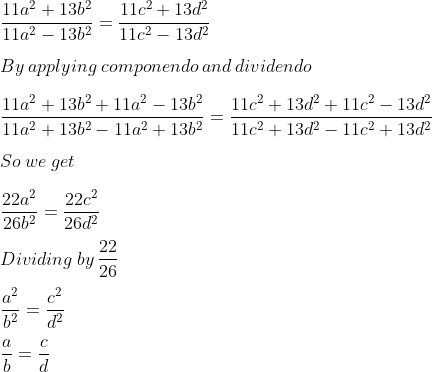Guru

# If (11a2 + 13b2) (11c2 – 13d2) = (11a2 – 13b2) (11c2 + 13d2), prove that a: b :: c: d.

• 0

This is a basic question from ML aggarwal book of class 10th, chapter 7, ratio and proportion., ICSE board

In this question we have been given that (11a2 + 13b2) (11c2 – 13d2) = (11a2 – 13b2) (11c2 + 13d2), and. We have to prove that a: b :: c: d.

Question 6, 7.3

Share

1. Solution:

It is given that

(11a2 + 13b2) (11c2 – 13d2) = (11a2 – 13b2) (11c2 + 13d2)

We can write it asTherefore, it is proved that a: b :: c: d.

• 0